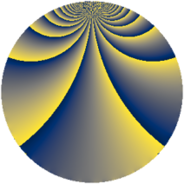# Properties

 Label 864.1.bdLevel $864$ Weight $1$ Character orbit 864.bd Rep. character $\chi_{864}(79,\cdot)$ Character field $\Q(\zeta_{18})$ Dimension $6$ Newform subspaces $1$ Sturm bound $144$ Trace bound $0$

# Related objects

## Defining parameters

 Level: $$N$$ $$=$$ $$864 = 2^{5} \cdot 3^{3}$$ Weight: $$k$$ $$=$$ $$1$$ Character orbit: $$[\chi]$$ $$=$$ 864.bd (of order $$18$$ and degree $$6$$) Character conductor: $$\operatorname{cond}(\chi)$$ $$=$$ $$216$$ Character field: $$\Q(\zeta_{18})$$ Newform subspaces: $$1$$ Sturm bound: $$144$$ Trace bound: $$0$$

## Dimensions

The following table gives the dimensions of various subspaces of $$M_{1}(864, [\chi])$$.

Total New Old
Modular forms 72 18 54
Cusp forms 24 6 18
Eisenstein series 48 12 36

The following table gives the dimensions of subspaces with specified projective image type.

$$D_n$$ $$A_4$$ $$S_4$$ $$A_5$$
Dimension 6 0 0 0

## Trace form

 $$6 q + O(q^{10})$$ $$6 q + 3 q^{11} + 3 q^{27} - 3 q^{33} - 3 q^{41} + 3 q^{43} - 6 q^{51} - 3 q^{57} - 6 q^{59} + 3 q^{67} + 3 q^{89} - 3 q^{97} + O(q^{100})$$

## Decomposition of $$S_{1}^{\mathrm{new}}(864, [\chi])$$ into newform subspaces

Label Dim. $$A$$ Field Image CM RM Traces $q$-expansion
$$a_2$$ $$a_3$$ $$a_5$$ $$a_7$$
864.1.bd.a $$6$$ $$0.431$$ $$\Q(\zeta_{18})$$ $$D_{9}$$ $$\Q(\sqrt{-2})$$ None $$0$$ $$0$$ $$0$$ $$0$$ $$q+\zeta_{18}q^{3}+\zeta_{18}^{2}q^{9}+(\zeta_{18}^{5}-\zeta_{18}^{6}+\cdots)q^{11}+\cdots$$

## Decomposition of $$S_{1}^{\mathrm{old}}(864, [\chi])$$ into lower level spaces

$$S_{1}^{\mathrm{old}}(864, [\chi]) \cong$$ $$S_{1}^{\mathrm{new}}(216, [\chi])$$$$^{\oplus 3}$$